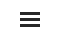# Appendix A: Index Construction Methodology

By Jesse Livermore+, Chris Meredith, Patrick O’Shaughnessy
May 2018

Sign up to receive a monthly email summary of both our research and the best of what we learned from others.
SUBSCRIBE

To build an index for an investment strategy, we model the strategy as if it were being implemented in an actual fund. We define the outstanding share count of the fund to be one, and then we track the aggregate fundamentals of the fund over time. Those fundamentals will already be represented on a per share basis, since the fund only has one share.

To illustrate, suppose that we want to build an equally-weighted index of 10 stocks. We start by specifying some arbitrary amount of money--say, \$1,000--to serve as the starting value of the index. We then take the money and distribute it equally across the 10 stocks, investing \$100 in each. The index will end up owning specific numbers of shares of each stock. Using those numbers, we can calculate per share metrics for the index. For example:

Price (Per Share): To calculate the price of the index at a given time, we multiply the price of each stock in the index by the number of shares that the index owns. The sum is the price of the index.

Earnings Per Share (EPS): To calculate the EPS of the index at a given time, we multiply the EPS of each company in the index by the number of shares that the index owns. The sum is the EPS of the index.

Price-to-Earnings (P/E) Ratio: To calculate the price-to-earnings (P/E) ratio of the index at a given time, we divide the price of the index by the EPS of the index. The result is the P/E ratio of the index.

To rebalance the index on the rebalancing date, we sell the old collection of companies that were in it, and use the proceeds to buy the new collection. By rebalancing the index in this way, we ensure that the price of the index and the market value contained within it remain constant through the rebalancing, a boundary condition that must be satisfied if the index is going to maintain its identity as the "same" index.

Most index construction methodologies extract dividends from the index when they are paid out, in the same way that they are extracted from the balance sheets of the underlying companies that pay them. This approach creates distortions when investment strategies that have different payout ratios are compared to each other. To eliminate these distortions, we reinvest all dividends internally, effectively converting them into share buybacks spread across the index. The benefit to this approach is that it puts investment strategies with different payout ratios on an equal footing for comparison. Dividends, buybacks and organic growth, which are equivalent and fungible sources of return, end up appearing as a single common "thing" that can be compared across different strategies: growth in fundamentals per share.